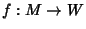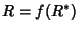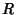## Change of Variables Theorem

A theorem which effectively describes how lengths, areas, volumes, and generalized-dimensional volumes (Contents) are distorted by Differentiable Functions. In particular, the change of variables theorem reduces the whole problem of figuring out the distortion of the content to understanding the infinitesimal distortion, i.e., the distortion of the Derivative (a linear Map), which is given by the linear Map's Determinant. Sois an Area-Preserving linear Map Iff, and in more generality, ifis any subset of, the Content of its image is given bytimes the Content of the original. The change of variables theorem takes this infinitesimal knowledge, and applies Calculus by breaking up the Domain into small pieces and adds up the change in Area, bit by bit.

The change of variable formula persists to the generality of Differential Forms on Manifolds, giving the formulaunder the conditions thatandare compact connected oriented Manifolds with nonempty boundaries,is a smooth map which is an orientation-preserving Diffeomorphism of the boundaries.

In 2-D, the explicit statement of the theorem isand in 3-D, it iswhereis the image of the original region,is the Jacobian, andis a global orientation-preserving Diffeomorphism ofand(which are open subsets of).

The change of variables theorem is a simple consequence of the Curl Theorem and a little de Rham Cohomology. The generalization to-D requires no additional assumptions other than the regularity conditions on the boundary.

Kaplan, W. Change of Variables in Integrals.'' §4.6 in Advanced Calculus, 3rd ed. Reading, MA: Addison-Wesley, pp. 238-245, 1984.